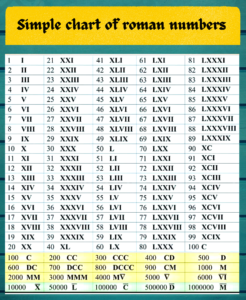June 28, 2022

# “What is L in Roman numbers”

## “What is L in Roman numbers”

Roman numbers  are the letters used by the Romans for the representation of cardinal numbers . They used 7 letters to write the Roman numerals . The 7 letters  represent the following value

I = 1

V = 5

X = 10

L = 50

C = 100

D = 500

and M = 1000

There are some specific rules to write Roman numerals . When a numeral is followed by a lower denomination numeral, the two are added together. The smaller numeral is subtracted from the greater if it is preceded by one of lower denomination. For example :  (i) VI = 6 (V + I), but IV = 4 (V – I)

(ii) LX= 50+10=60  but XL =  50-10=40

Multiple of thousands are indicated by a bar over the letters.  Example :

= 5000  and 9000=   etc.

### Chart of Roman Numerals: